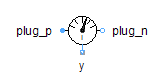Linear Permanent Magnet - MapleSim Help

Linear Permanent Magnet

Permanent Magnet with linear characteristicDescription The Linear Permanent Magnet component is a flux tube of fixed length and cross-sectional area that models a linear permanent magnet using material data. The Hard Material parameter specifies the name of a record of class Hard Magnetic Material, which defines the magnetic material. A record of that class, with the corresponding name, must exist in the model, either at the same or higher level. Records of typical materials are in the palette Magnetic > Material > Hard. The Use default material boolean parameter, when true, sets the name of the Hard Material parameter to HardMaterial1, which corresponds to the default name assigned to a record of the appropriate class.  To use a different name, uncheck the box and enter the name for the parameter that appears.Equations $\mathrm{\Phi }={\mathrm{\Phi }}_{p}=-{\mathrm{\Phi }}_{n}=BA$ ${V}_{m}={V}_{{m}_{p}}-{V}_{{m}_{n}}=H\ell$ $B={\mathrm{\mu }}_{r}{\mathrm{\mu }}_{0}H-{B}_{r}$Variables

 Name Units Description Modelica ID ${V}_{m}$ $A$ Magnetic potential difference between both ports V_m $\mathrm{\Phi }$ $\mathrm{Wb}$ Magnetic flux from port_p to port_n Phi $H$ $\frac{A}{m}$ Magnetic field intensity H $B$ $T$ Magnetic flux density B ${B}_{r}$ $T$ Residual magnetic flux density of material mat.B_r ${\mathrm{\mu }}_{r}$ $1$ Relative permeability of material mat.mu_rConnections

 Name Description Modelica ID ${\mathrm{port}}_{p}$ Positive magnetic port port_p ${\mathrm{port}}_{n}$ Negative magnetic port port_nParametersMaterial

 Name Default Units Description Modelica ID Hard Material  Magnetic Material (hard) mat Use default material $\mathrm{true}$ True (checked) uses hardMaterial1 as the name for the Hard Material parameter useDefaultMaterial

 hardMaterial1Fixed Geometry

 Name Default Units Description Modelica ID $\ell$ $0.1$ $m$ Length in direction of flux l $A$ $1·{10}^{-4}$ ${m}^{2}$ Area of cross section AModelica Standard Library The component described in this topic is from the Modelica Standard Library. To view the original documentation, which includes author and copyright information, click here.